#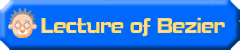## 11.It traces out a sketch by the Bezier curve. ( Procedure 1 of the trace )

It traces out the image which was actually arranged as the template using Adobe Illustrator.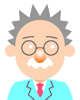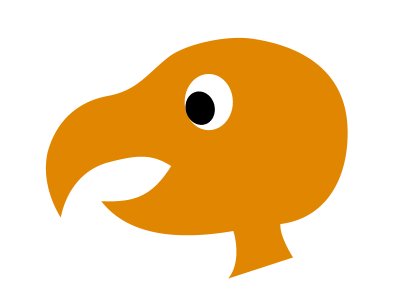( Figure 1 )

This is the character which was made for the explanation ( Figure 1 ).It is places such as the buzzard.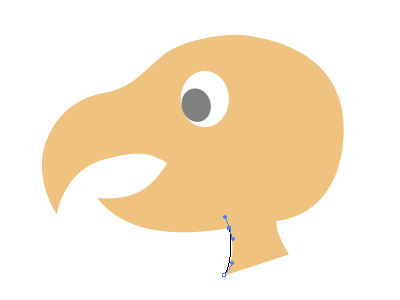( Figure 2 )

It traces out the sketch of the image which was arranged as the template from the underside in Adobe Illustrator.( Figure 2 )

It chooses Pen tool and it begins to draw it.Because it adjusts a Bezier curve from behind, without becoming nervous at first, it draws an approximate form only and it is OK.
( It may draw a path as in the sketch of course in there is a crack between the sketch and the path but it is OK now in this. ).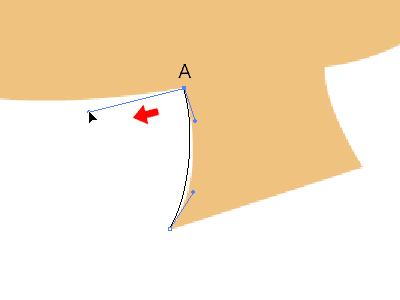( Figure 3 )

The part in the corner is on anchor points "A" while pushing [Option] key (Mac)/[Alt] key (Win), and pushes, and drags the ( left ) button of the mouse below, to be few just as it is on the left and divides a control handle.( Figure 3 )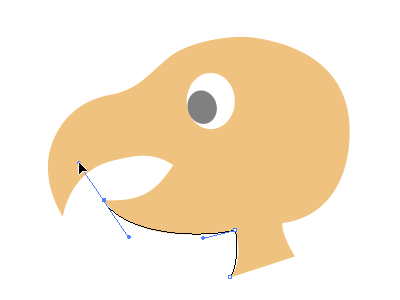( Figure 4 )

It extends a Bezier curve to the end of the bill.( Figure 4 )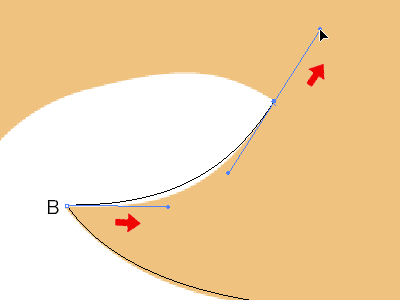( Figure 5 )

It turns around from the Bezier curve and it is in the bill boiling below.( Figure 5 )
It pushes the ( left ) button of the mouse on anchor points "B" while pushing [Option] key (Mac)/[Alt] key (Win), that the part at the end of this turning bill, too, is same ( as figure 3 ) and it is a drag on the right.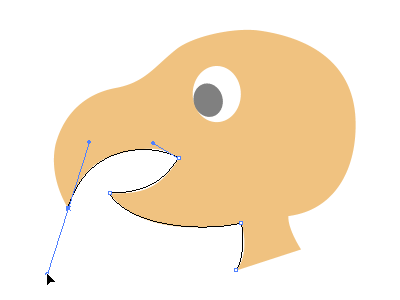( Figure 6 )

This time, it turns around from the Bezier curve, being in the mouth, and it turns around once again at the end of the bill from it and it draws a bill above.( Figure 6 )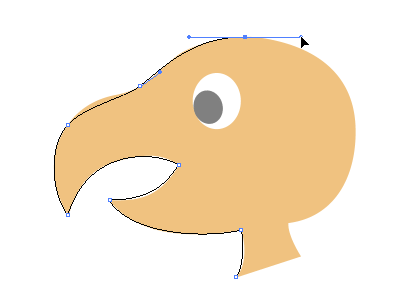( Figure 7 )

It draws to the top of the head.( Figure 7 )
With the bill, it strikes an anchor points for the convexity and the changing hit with the concavity, the head in the convexity, at the base.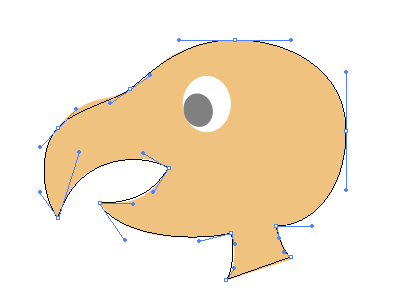( Figure 8 )

When closing a Bezier curve even if it got off just as it is, the form which becomes basic was made.( Figure 8 )

With the following 12nd lecture, it adjusts in this Bezier curve.